Next: Method rodriguez Up: Implementation Previous: Implementation   Contents

## Method ambiguity

This method yields first the heights of the (line,pixel) and the position P(x,y,z). In this manner the geocoding can be done in the same step. by converting P(x,y,z) (known h) to phi,lambda. If there is a trend in the height this has to be removed first, e.g. by using tiepoints. This means the computed phi and lambda matrices are not correct anymore.

With the baseline defined as in Annex D the following equations hold.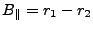(1)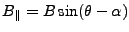(2)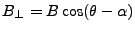(3)

Note the sign, must be minus.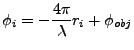(4)

Content of unwrapped interferogram (never mind the -phiR).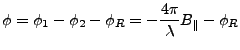(5)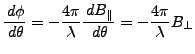(6)

Geometric equation: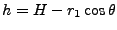(7)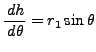(8)

Height ambiguity: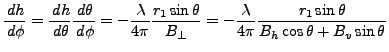(9)

Final equation to convert phase to height: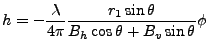(10)

The procedure to compute the height is as follows (note that computation is skipped if unwrapping went wrong, indicated by NaN (not a number) in the unwrapped interferogram.)

1. for all lines
1. .i1. compute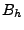and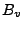(by computing h for middle pixel, then compute baseline).
2. .i2. hcurrent = 0
3. for all pixels
1. .j1. if value unwrapped phase equals NaN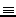-999 then goto next pixel
2. .j2. compute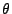(corresponding to h)
3. .j3. hlast=hcurrent, compute hcurrent with formula
4. if ((hlast - hcurrent )k) then goto .j2.Next: Method rodriguez Up: Implementation Previous: Implementation   Contents
Leijen 2009-04-14